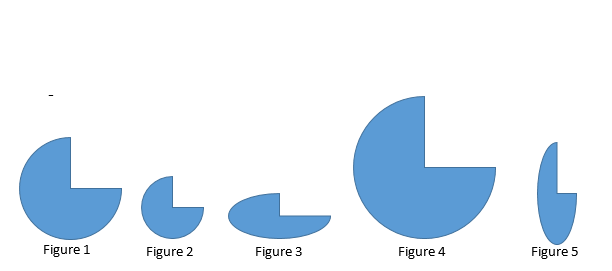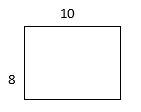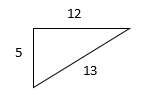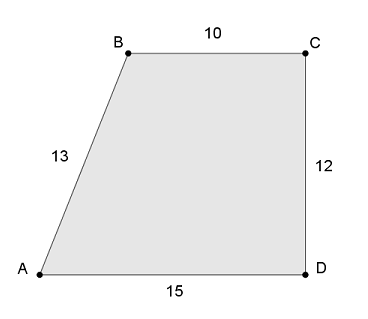Match Fishtank is now Fishtank Learning!

# Transformations and Angle Relationships

## Objective

Define a dilation as a non-rigid transformation, and understand the impact of scale factor.

## Common Core Standards

### Core Standards

?

• 8.G.A.4 — Understand that a two-dimensional figure is similar to another if the second can be obtained from the first by a sequence of rotations, reflections, translations, and dilations; given two similar two-dimensional figures, describe a sequence that exhibits the similarity between them.

?

• 7.G.A.1

• 7.RP.A.2

## Criteria for Success

?

1. Understand a dilation as a non-rigid transformation that proportionally changes the size of a figure while maintaining the same shape.
2. Understand that corresponding angle measures in dilated figures are congruent.
3. Understand that a scale factor greater than 1 will enlarge a figure and a scale factor less than 1 will shrink a figure.
4. Sketch and identify images of dilated figures with a described scale factor.

## Tips for Teachers

?

• Students should be familiar with the concept of a scale drawing from seventh grade; however, it will be new for them to apply this concept as a transformation.
• This video, Understanding Dilations, by PBS Learning Media introduces the concept of dilation and includes animation that can be helpful for students to visualize the movements.
• The following materials are useful for this lesson: patty paper (or transparency paper), protractors

#### Remote Learning Guidance

If you need to adapt or shorten this lesson for remote learning, we suggest prioritizing Anchor Problems 2 and 3 (benefit from worked examples). Find more guidance on adapting our math curriculum for remote learning here.

#### Fishtank Plus

• Problem Set
• Student Handout Editor
• Vocabulary Package

## Anchor Problems

?

### Problem 1

Several figures are shown below.1.  What do you notice? What do you wonder about the relationships between the shapes?
2. A dilation is a transformation that enlarges or shrinks a figure in a proportional way so that the shape remains the same. Where do you see evidence of a dilation in the figures?

### Problem 2

Triangle ${ABC}$ is dilated to create similar triangle ${DEF}$.1. Indicate the corresponding angles in the diagram. What is the relationship between corresponding angles?
2. Name the corresponding sides in the diagram. What is the relationship between corresponding side lengths?

### Problem 3

Sketch an image of each figure after the dilations described below. The figures do not need to be drawn exactly to size but should include the lengths of the sides.

1. Rectangle ${ABCD}$ is dilated by a scale factor of ${\frac{1}{2}}$.1. Triangle ${LMN}$ is dilated by a scale factor of 3.## Problem Set

?The following resources include problems and activities aligned to the objective of the lesson that can be used to create your own problem set.

• Examples where students are given an original figure and then multiple other dilated figures; students identify which figures are dilations (proportional) and which are not dilations; be sure to include images with scale factor equal to 1
• Examples where students identify corresponding line segments and angles between a figure and a dilation; include examples where the dilated image is in a different orientation from the original

?

Trapezoid ${ABCD}$, shown below, is dilated by a scale factor of ${\frac{1}{4}}$. Angle $D$ is a right angle.1. Which statements are true? Select all that apply.

a.     ${\overline{C'D'}}$ will be ${48}$ units long.

b.     ${\overline{B'C'}}$ will be ${2\frac{1}{2}}$ units long.

c.     ${{\overline{A'D'}}}$ will be ${15}$ units long.

d.     The measure of ${{\angle B}'}$ will be ${\frac{1}{4}}$ the measurement of ${\angle B}$.

e.     ${\angle D'}$ will be a right angle.

f.     ${\overline {B'C'}}$ will be parallel to ${{\overline{A'D'}}}$.

g.     Figure ${A'B'C'D'}$ will be a trapezoid.

2. Explain your response to answer choices (b) and (d). Why did you decide that those answer choices were true or false?

?